# Mean State

Period Mean (original grids) [Watt m-2]
Model Period Mean (intersection) [Watt m-2]
Model Period Mean (complement) [Watt m-2]
Benchmark Period Mean (intersection) [Watt m-2]
Benchmark Period Mean (complement) [Watt m-2]
Bias [Watt m-2]
RMSE [Watt m-2]
Phase Shift [months]
Bias Score 
RMSE Score 
Seasonal Cycle Score 
Spatial Distribution Score 
Interannual Variability Score 
Overall Score 
Benchmark [-] 397.
CLM4 [-] 395. 395. 0.00 396. 408. -0.219 6.93 0.212 0.82 0.50 0.97 1.0 0.75 0.76
CLM4.5 [-] 395. 395. 0.00 396. 408. -0.219 6.93 0.212 0.82 0.50 0.97 1.0 0.75 0.76
CLM5 [-] 395. 395. 0.00 396. 408. -0.219 6.93 0.212 0.82 0.50 0.97 1.0 0.75 0.76
Period Mean (original grids) [Watt m-2]
Model Period Mean (intersection) [Watt m-2]
Model Period Mean (complement) [Watt m-2]
Benchmark Period Mean (intersection) [Watt m-2]
Benchmark Period Mean (complement) [Watt m-2]
Bias [Watt m-2]
RMSE [Watt m-2]
Phase Shift [months]
Bias Score 
RMSE Score 
Seasonal Cycle Score 
Spatial Distribution Score 
Interannual Variability Score 
Overall Score 
Benchmark [-] 384.
CLM4 [-] 384. 384. 0.00 384. 397. -0.0965 9.48 0.181 0.90 0.69 0.99 1.0 0.91 0.86
CLM4.5 [-] 384. 384. 0.00 384. 397. -0.0965 9.48 0.181 0.90 0.69 0.99 1.0 0.91 0.86
CLM5 [-] 384. 384. 0.00 384. 397. -0.0965 9.48 0.181 0.90 0.69 0.99 1.0 0.91 0.86
Period Mean (original grids) [Watt m-2]
Model Period Mean (intersection) [Watt m-2]
Model Period Mean (complement) [Watt m-2]
Benchmark Period Mean (intersection) [Watt m-2]
Benchmark Period Mean (complement) [Watt m-2]
Bias [Watt m-2]
RMSE [Watt m-2]
Phase Shift [months]
Bias Score 
RMSE Score 
Seasonal Cycle Score 
Spatial Distribution Score 
Interannual Variability Score 
Overall Score 
Benchmark [-] 362.
CLM4 [-] 359. 359. 0.00 361. 382. 0.0495 8.96 0.237 0.88 0.70 0.97 1.0 0.85 0.85
CLM4.5 [-] 359. 359. 0.00 361. 382. 0.0495 8.96 0.237 0.88 0.70 0.97 1.0 0.85 0.85
CLM5 [-] 359. 359. 0.00 361. 382. 0.0495 8.96 0.237 0.88 0.70 0.97 1.0 0.85 0.85
Period Mean (original grids) [Watt m-2]
Model Period Mean (intersection) [Watt m-2]
Model Period Mean (complement) [Watt m-2]
Benchmark Period Mean (intersection) [Watt m-2]
Benchmark Period Mean (complement) [Watt m-2]
Bias [Watt m-2]
RMSE [Watt m-2]
Phase Shift [months]
Bias Score 
RMSE Score 
Seasonal Cycle Score 
Spatial Distribution Score 
Interannual Variability Score 
Overall Score 
Benchmark [-] 239.
CLM4 [-] 236. 236. 0.00 238. 251. 0.254 9.46 0.0140 0.96 0.83 1.0 1.0 0.89 0.92
CLM4.5 [-] 236. 236. 0.00 238. 251. 0.254 9.46 0.0140 0.96 0.83 1.0 1.0 0.89 0.92
CLM5 [-] 236. 236. 0.00 238. 251. 0.254 9.46 0.0140 0.96 0.83 1.0 1.0 0.89 0.92
Period Mean (original grids) [Watt m-2]
Model Period Mean (intersection) [Watt m-2]
Model Period Mean (complement) [Watt m-2]
Benchmark Period Mean (intersection) [Watt m-2]
Benchmark Period Mean (complement) [Watt m-2]
Bias [Watt m-2]
RMSE [Watt m-2]
Phase Shift [months]
Bias Score 
RMSE Score 
Seasonal Cycle Score 
Spatial Distribution Score 
Interannual Variability Score 
Overall Score 
Benchmark [-] 411.
CLM4 [-] 408. 408. 0.00 410. 415. 0.0344 5.44 0.531 0.67 0.40 0.92 0.99 0.74 0.69
CLM4.5 [-] 408. 408. 0.00 410. 415. 0.0344 5.44 0.531 0.67 0.40 0.92 0.99 0.74 0.69
CLM5 [-] 408. 408. 0.00 410. 415. 0.0344 5.44 0.531 0.67 0.40 0.92 0.99 0.74 0.69
Period Mean (original grids) [Watt m-2]
Model Period Mean (intersection) [Watt m-2]
Model Period Mean (complement) [Watt m-2]
Benchmark Period Mean (intersection) [Watt m-2]
Benchmark Period Mean (complement) [Watt m-2]
Bias [Watt m-2]
RMSE [Watt m-2]
Phase Shift [months]
Bias Score 
RMSE Score 
Seasonal Cycle Score 
Spatial Distribution Score 
Interannual Variability Score 
Overall Score 
Benchmark [-] 264.
CLM4 [-] 264. 263. 0.00 264. 277. 0.0194 8.45 0.00704 0.97 0.86 1.0 1.0 0.81 0.92
CLM4.5 [-] 264. 263. 0.00 264. 277. 0.0194 8.45 0.00704 0.97 0.86 1.0 1.0 0.81 0.92
CLM5 [-] 264. 263. 0.00 264. 277. 0.0194 8.45 0.00704 0.97 0.86 1.0 1.0 0.81 0.92
Period Mean (original grids) [Watt m-2]
Model Period Mean (intersection) [Watt m-2]
Model Period Mean (complement) [Watt m-2]
Benchmark Period Mean (intersection) [Watt m-2]
Benchmark Period Mean (complement) [Watt m-2]
Bias [Watt m-2]
RMSE [Watt m-2]
Phase Shift [months]
Bias Score 
RMSE Score 
Seasonal Cycle Score 
Spatial Distribution Score 
Interannual Variability Score 
Overall Score 
Benchmark [-] 294.
CLM4 [-] 291. 291. 0.00 293. 336. 0.277 9.99 0.0242 0.94 0.82 1.0 1.0 0.78 0.90
CLM4.5 [-] 291. 291. 0.00 293. 336. 0.277 9.99 0.0242 0.94 0.82 1.0 1.0 0.78 0.90
CLM5 [-] 291. 291. 0.00 293. 336. 0.277 9.99 0.0242 0.94 0.82 1.0 1.0 0.78 0.90
Period Mean (original grids) [Watt m-2]
Model Period Mean (intersection) [Watt m-2]
Model Period Mean (complement) [Watt m-2]
Benchmark Period Mean (intersection) [Watt m-2]
Benchmark Period Mean (complement) [Watt m-2]
Bias [Watt m-2]
RMSE [Watt m-2]
Phase Shift [months]
Bias Score 
RMSE Score 
Seasonal Cycle Score 
Spatial Distribution Score 
Interannual Variability Score 
Overall Score 
Benchmark [-] 379.
CLM4 [-] 371. 372. 0.00 377. 410. -0.454 9.29 0.100 0.88 0.75 0.99 1.0 0.88 0.87
CLM4.5 [-] 371. 372. 0.00 377. 410. -0.454 9.29 0.100 0.88 0.75 0.99 1.0 0.88 0.87
CLM5 [-] 371. 372. 0.00 377. 410. -0.454 9.29 0.100 0.88 0.75 0.99 1.0 0.88 0.87
Period Mean (original grids) [Watt m-2]
Model Period Mean (intersection) [Watt m-2]
Model Period Mean (complement) [Watt m-2]
Benchmark Period Mean (intersection) [Watt m-2]
Benchmark Period Mean (complement) [Watt m-2]
Bias [Watt m-2]
RMSE [Watt m-2]
Phase Shift [months]
Bias Score 
RMSE Score 
Seasonal Cycle Score 
Spatial Distribution Score 
Interannual Variability Score 
Overall Score 
Benchmark [-] 244.
CLM4 [-] 243. 243. 0.00 244. 248. 0.0310 8.41 0.00100 0.98 0.84 1.0 1.0 0.88 0.92
CLM4.5 [-] 243. 243. 0.00 244. 248. 0.0310 8.41 0.00100 0.98 0.84 1.0 1.0 0.88 0.92
CLM5 [-] 243. 243. 0.00 244. 248. 0.0310 8.41 0.00100 0.98 0.84 1.0 1.0 0.88 0.92
Period Mean (original grids) [Watt m-2]
Model Period Mean (intersection) [Watt m-2]
Model Period Mean (complement) [Watt m-2]
Benchmark Period Mean (intersection) [Watt m-2]
Benchmark Period Mean (complement) [Watt m-2]
Bias [Watt m-2]
RMSE [Watt m-2]
Phase Shift [months]
Bias Score 
RMSE Score 
Seasonal Cycle Score 
Spatial Distribution Score 
Interannual Variability Score 
Overall Score 
Benchmark [-] 351.
CLM4 [-] 350. 350. 0.00 350. 359. 0.0571 7.85 0.104 0.93 0.78 0.99 1.0 0.91 0.90
CLM4.5 [-] 350. 350. 0.00 350. 359. 0.0571 7.85 0.104 0.93 0.78 0.99 1.0 0.91 0.90
CLM5 [-] 350. 350. 0.00 350. 359. 0.0571 7.85 0.104 0.93 0.78 0.99 1.0 0.91 0.90
Period Mean (original grids) [Watt m-2]
Model Period Mean (intersection) [Watt m-2]
Model Period Mean (complement) [Watt m-2]
Benchmark Period Mean (intersection) [Watt m-2]
Benchmark Period Mean (complement) [Watt m-2]
Bias [Watt m-2]
RMSE [Watt m-2]
Phase Shift [months]
Bias Score 
RMSE Score 
Seasonal Cycle Score 
Spatial Distribution Score 
Interannual Variability Score 
Overall Score 
Benchmark [-] 308.
CLM4 [-] 304. 304. 0.00 307. 324. 0.0546 8.06 0.0712 0.93 0.78 1.0 1.0 0.74 0.87
CLM4.5 [-] 304. 304. 0.00 307. 324. 0.0546 8.06 0.0712 0.93 0.78 1.0 1.0 0.74 0.87
CLM5 [-] 304. 304. 0.00 307. 324. 0.0546 8.06 0.0712 0.93 0.78 1.0 1.0 0.74 0.87
Period Mean (original grids) [Watt m-2]
Model Period Mean (intersection) [Watt m-2]
Model Period Mean (complement) [Watt m-2]
Benchmark Period Mean (intersection) [Watt m-2]
Benchmark Period Mean (complement) [Watt m-2]
Bias [Watt m-2]
RMSE [Watt m-2]
Phase Shift [months]
Bias Score 
RMSE Score 
Seasonal Cycle Score 
Spatial Distribution Score 
Interannual Variability Score 
Overall Score 
Benchmark [-] 344.
CLM4 [-] 308. 308. 0.00 311. 360. -0.0963 9.16 0.113 0.89 0.72 0.99 1.0 0.84 0.86
CLM4.5 [-] 308. 308. 0.00 311. 360. -0.0963 9.16 0.113 0.89 0.72 0.99 1.0 0.84 0.86
CLM5 [-] 308. 308. 0.00 311. 360. -0.0963 9.16 0.113 0.89 0.72 0.99 1.0 0.84 0.86
Period Mean (original grids) [Watt m-2]
Model Period Mean (intersection) [Watt m-2]
Model Period Mean (complement) [Watt m-2]
Benchmark Period Mean (intersection) [Watt m-2]
Benchmark Period Mean (complement) [Watt m-2]
Bias [Watt m-2]
RMSE [Watt m-2]
Phase Shift [months]
Bias Score 
RMSE Score 
Seasonal Cycle Score 
Spatial Distribution Score 
Interannual Variability Score 
Overall Score 
Benchmark [-] 343.
CLM4 [-] 342. 342. 0.00 343. 360. 0.000187 11.3 0.0949 0.92 0.72 0.99 1.0 0.89 0.87
CLM4.5 [-] 342. 342. 0.00 343. 360. 0.000187 11.3 0.0949 0.92 0.72 0.99 1.0 0.89 0.87
CLM5 [-] 342. 342. 0.00 343. 360. 0.000187 11.3 0.0949 0.92 0.72 0.99 1.0 0.89 0.87
Period Mean (original grids) [Watt m-2]
Model Period Mean (intersection) [Watt m-2]
Model Period Mean (complement) [Watt m-2]
Benchmark Period Mean (intersection) [Watt m-2]
Benchmark Period Mean (complement) [Watt m-2]
Bias [Watt m-2]
RMSE [Watt m-2]
Phase Shift [months]
Bias Score 
RMSE Score 
Seasonal Cycle Score 
Spatial Distribution Score 
Interannual Variability Score 
Overall Score 
Benchmark [-] 244.
CLM4 [-] 243. 243. 0.00 243. 253. -0.0103 8.60 0.0247 0.97 0.83 1.0 1.0 0.88 0.92
CLM4.5 [-] 243. 243. 0.00 243. 253. -0.0103 8.60 0.0247 0.97 0.83 1.0 1.0 0.88 0.92
CLM5 [-] 243. 243. 0.00 243. 253. -0.0103 8.60 0.0247 0.97 0.83 1.0 1.0 0.88 0.92
Period Mean (original grids) [Watt m-2]
Model Period Mean (intersection) [Watt m-2]
Model Period Mean (complement) [Watt m-2]
Benchmark Period Mean (intersection) [Watt m-2]
Benchmark Period Mean (complement) [Watt m-2]
Bias [Watt m-2]
RMSE [Watt m-2]
Phase Shift [months]
Bias Score 
RMSE Score 
Seasonal Cycle Score 
Spatial Distribution Score 
Interannual Variability Score 
Overall Score 
Benchmark [-] 374.
CLM4 [-] 362. 362. 0.00 369. 398. 0.0604 9.12 0.254 0.82 0.68 0.97 1.0 0.87 0.84
CLM4.5 [-] 362. 362. 0.00 369. 398. 0.0604 9.12 0.254 0.82 0.68 0.97 1.0 0.87 0.84
CLM5 [-] 362. 362. 0.00 369. 398. 0.0604 9.12 0.254 0.82 0.68 0.97 1.0 0.87 0.84
Period Mean (original grids) [Watt m-2]
Model Period Mean (intersection) [Watt m-2]
Model Period Mean (complement) [Watt m-2]
Benchmark Period Mean (intersection) [Watt m-2]
Benchmark Period Mean (complement) [Watt m-2]
Bias [Watt m-2]
RMSE [Watt m-2]
Phase Shift [months]
Bias Score 
RMSE Score 
Seasonal Cycle Score 
Spatial Distribution Score 
Interannual Variability Score 
Overall Score 
Benchmark [-] 332.
CLM4 [-] 328. 328. 0.00 331. 370. -0.0972 7.51 0.0275 0.95 0.84 1.0 1.0 0.84 0.91
CLM4.5 [-] 328. 328. 0.00 331. 370. -0.0972 7.51 0.0275 0.95 0.84 1.0 1.0 0.84 0.91
CLM5 [-] 328. 328. 0.00 331. 370. -0.0972 7.51 0.0275 0.95 0.84 1.0 1.0 0.84 0.91
Period Mean (original grids) [Watt m-2]
Model Period Mean (intersection) [Watt m-2]
Model Period Mean (complement) [Watt m-2]
Benchmark Period Mean (intersection) [Watt m-2]
Benchmark Period Mean (complement) [Watt m-2]
Bias [Watt m-2]
RMSE [Watt m-2]
Phase Shift [months]
Bias Score 
RMSE Score 
Seasonal Cycle Score 
Spatial Distribution Score 
Interannual Variability Score 
Overall Score 
Benchmark [-] 277.
CLM4 [-] 274. 275. 0.00 276. 298. -0.0815 7.66 0.0260 0.95 0.84 1.0 1.0 0.83 0.91
CLM4.5 [-] 274. 275. 0.00 276. 298. -0.0815 7.66 0.0260 0.95 0.84 1.0 1.0 0.83 0.91
CLM5 [-] 274. 275. 0.00 276. 298. -0.0815 7.66 0.0260 0.95 0.84 1.0 1.0 0.83 0.91
Period Mean (original grids) [Watt m-2]
Model Period Mean (intersection) [Watt m-2]
Model Period Mean (complement) [Watt m-2]
Benchmark Period Mean (intersection) [Watt m-2]
Benchmark Period Mean (complement) [Watt m-2]
Bias [Watt m-2]
RMSE [Watt m-2]
Phase Shift [months]
Bias Score 
RMSE Score 
Seasonal Cycle Score 
Spatial Distribution Score 
Interannual Variability Score 
Overall Score 
Benchmark [-] 296.
CLM4 [-] 293. 294. 0.00 295. 324. -0.178 9.51 0.0536 0.91 0.77 1.0 1.0 0.79 0.87
CLM4.5 [-] 293. 294. 0.00 295. 324. -0.178 9.51 0.0536 0.91 0.77 1.0 1.0 0.79 0.87
CLM5 [-] 293. 294. 0.00 295. 324. -0.178 9.51 0.0536 0.91 0.77 1.0 1.0 0.79 0.87
Period Mean (original grids) [Watt m-2]
Model Period Mean (intersection) [Watt m-2]
Model Period Mean (complement) [Watt m-2]
Benchmark Period Mean (intersection) [Watt m-2]
Benchmark Period Mean (complement) [Watt m-2]
Bias [Watt m-2]
RMSE [Watt m-2]
Phase Shift [months]
Bias Score 
RMSE Score 
Seasonal Cycle Score 
Spatial Distribution Score 
Interannual Variability Score 
Overall Score 
Benchmark [-] 406.
CLM4 [-] 405. 405. 0.00 405. 410. -0.270 6.26 0.342 0.80 0.50 0.95 1.0 0.82 0.76
CLM4.5 [-] 405. 405. 0.00 405. 410. -0.270 6.26 0.342 0.80 0.50 0.95 1.0 0.82 0.76
CLM5 [-] 405. 405. 0.00 405. 410. -0.270 6.26 0.342 0.80 0.50 0.95 1.0 0.82 0.76
Period Mean (original grids) [Watt m-2]
Model Period Mean (intersection) [Watt m-2]
Model Period Mean (complement) [Watt m-2]
Benchmark Period Mean (intersection) [Watt m-2]
Benchmark Period Mean (complement) [Watt m-2]
Bias [Watt m-2]
RMSE [Watt m-2]
Phase Shift [months]
Bias Score 
RMSE Score 
Seasonal Cycle Score 
Spatial Distribution Score 
Interannual Variability Score 
Overall Score 
Benchmark [-] 376.
CLM4 [-] 376. 375. 0.00 376. 374. -0.175 9.06 0.144 0.89 0.66 0.98 1.0 0.84 0.84
CLM4.5 [-] 376. 375. 0.00 376. 374. -0.175 9.06 0.144 0.89 0.66 0.98 1.0 0.84 0.84
CLM5 [-] 376. 375. 0.00 376. 374. -0.175 9.06 0.144 0.89 0.66 0.98 1.0 0.84 0.84
Period Mean (original grids) [Watt m-2]
Model Period Mean (intersection) [Watt m-2]
Model Period Mean (complement) [Watt m-2]
Benchmark Period Mean (intersection) [Watt m-2]
Benchmark Period Mean (complement) [Watt m-2]
Bias [Watt m-2]
RMSE [Watt m-2]
Phase Shift [months]
Bias Score 
RMSE Score 
Seasonal Cycle Score 
Spatial Distribution Score 
Interannual Variability Score 
Overall Score 
Benchmark [-] 307.
CLM4 [-] 301. 301. 0.00 303. 340. 1.13 13.5 0.185 0.72 0.58 0.98 1.0 0.75 0.77
CLM4.5 [-] 301. 301. 0.00 303. 340. 1.13 13.5 0.185 0.72 0.58 0.98 1.0 0.75 0.77
CLM5 [-] 301. 301. 0.00 303. 340. 1.13 13.5 0.185 0.72 0.58 0.98 1.0 0.75 0.77

# Temporally integrated period mean

BENCHMARK MEAN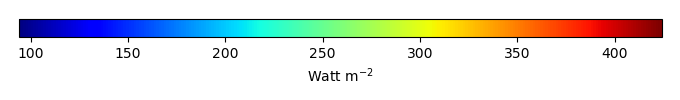MODEL MEANBIAS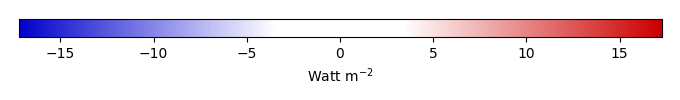BIAS SCORERMSE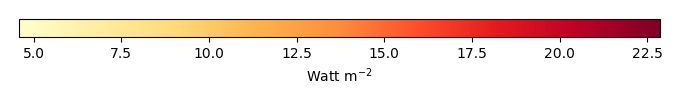RMSE SCOREBENCHMARK INTERANNUAL VARIABILITY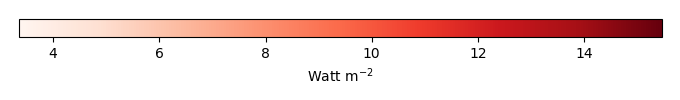MODEL INTERANNUAL VARIABILITYINTERANNUAL VARIABILITY SCOREBENCHMARK MAX MONTHMODEL MAX MONTHDIFFERENCE IN MAX MONTH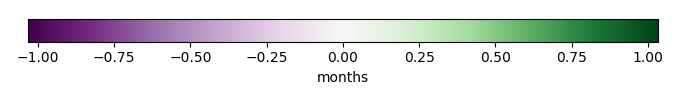SEASONAL CYCLE SCORESPATIAL TAYLOR DIAGRAMMODEL COLORS# Spatially integrated regional mean

MODEL COLORSREGIONAL MEANANNUAL CYCLEMONTHLY ANOMALYANNUAL CYCLE# All Models

BenchmarkCLM4CLM4.5CLM5# Data Information

creation_date: Mon Jun 30 23:41:07 PDT 2014

source_file: This product is generated from monthly 1 degree GEWEX SRB Radiation observations